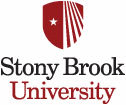MAT 534:
Fall 2020

## Welcome to MAT 534

Description: Groups: normal subgroups, quotient groups, Lagrange's theorem, class formula, finite p-groups and solvable groups, Sylow's theorems, finitely generated abelian groups. Rings and modules: subrings, fields, prime and maximal ideals, quotient rings, ID's, PID's, UFD's, polynomial rings, field of fractions, the Wedderburn theorem, Hilbert basis theorem, finitely generated modules over a PID. Vector spaces: basis, linear maps and matrices, dual spaces, determinants, eigenvalues and vectors, inner products, spectral theorem for normal operators.

Schedule:  TuTh 1:15pm- 2:35pm Javits Lectr 101